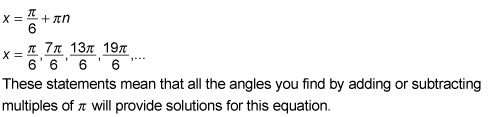##### Trigonometry Workbook For DummiesThe simplest type of trigonometry equation is the one that you can immediately rewrite as an inverse in order to determine the solutions. Some examples of these types of equations include: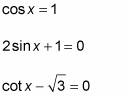To solve cos x = 1, follow these steps:

1. Rewrite the equation as an inverse function equation.

x = cos1(1)

2. List the solutions for values of x when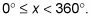x = 0°

The only time that the cosine is equal to 1 is when the angle, or input, is 0 degrees. The terminal sides of angles of 0 and 360 degrees are the same, so you don’t have to list the angle measure twice.

3. List all the solutions in general.

x = 0° + 360°n

Steps 2 and 3 illustrate the different ways that you can write the answers: either as a few within a certain interval, or as all that are possible, with a rule to describe them.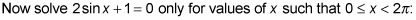1. Rewrite the equation as an inverse function equation.

First, subtract 1 from each side; then divide each side by 2.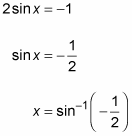2. List the solutions. When you use a Trig Function Table to find the angles that work, you find that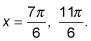The following example involves a reciprocal function. Your best bet is to begin by using a reciprocal identity and changing the problem.

Solve the equation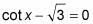for all the values of x, in radians, that satisfy it:

1. Solve for the trig function by adding the radical value to each side.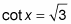2. Use the reciprocal identity and the reciprocal of the number to change to the tangent function and then multiply both parts of the fraction by the denominator to get rid of the radical.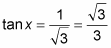3. Rewrite the equation as an inverse function equation.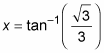4. Write the general statements that give all the solutions.